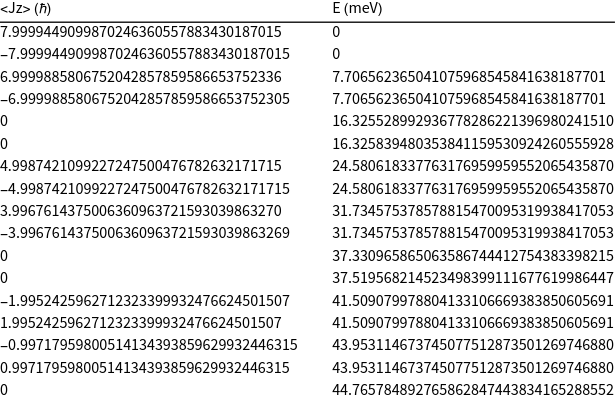GROUP THEORY TUTORIAL

# Crystal Field Theory – Stevens Operators and Ho/Pt(111)

We show the formulation of effective crystal field Hamiltonians using Stevens operator equivalents [Proc. Phys Soc Sect A 65, 209 (1952)], on the example of single Ho atoms on a clean Pt(111) surface. This system was shown to exhibit localized and well-defined magnetic moments with lifetimes of the order of minutes [Nature 503, 242246 (2013)]. Pt crystallizes in an fcc lattice and hence, the (111) surface exhibits a triangular symmetry with the symmetry group C3v.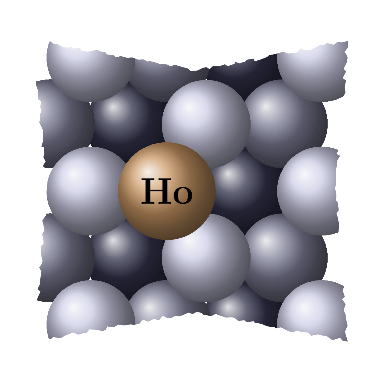GTCrystalField gives the crystal field Hamiltonian for a certain group up to a maximal angular momentum quantum number. GTStevensOperator calculates matrix elements over Stevens operator equivalents
Qualitative analysis. We start with a qualitative analysis of the system. Ho is a rare earth atom with 10 electrons in the f-shell [J.Phys. : Condens.Matter 18 6329 (2006)]. The f-orbitals are spin-orbit split into orbitals with j = 5/2 and j = 7/2, with the j = 5/2 orbital being lower in energy. According to Hunds rule, 6 electrons occupy the j = 5/2 orbital while the remaining 4 electrons occupy the j = 7/2 level with the magnetic quantum numbers m = 7/2, m = 5/2, m = 3/2, m = 1/2. Hence, as a result, a total angular momentum of j = 8 (7/2 + 5/2 + 3/2 + 1/2) arises for the ground state multiplet. We discuss the splitting of this multiplet if the symmetry is lowered to a trigonal symmetry by means of representation theory. C3v is installed using the command GTInstallGroup and it' s character table is obtained using GTCharacterTable.
 In:=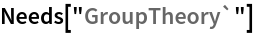In:=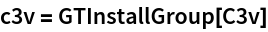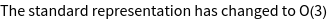Out=In:=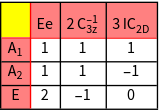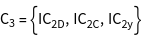Without the Pt surface, Ho would have an O(3) symmetry where the irreducible representations are denoted by the angular momentum quantum number. The characters of the irreducible representation with j = 8 for the symmetry elements in the three classes of C3v are obtained using GTAngularMomentumChars. This representation is reducible if the symmetry is lowered to C3v, where the corresponding decomposition into irreducible representations of C3v is obtained using GTIrep. The 2*j + 1 - dimensional (17 - dimensional) angular momentum representation decomposes into 3 times A1, 2 times A2, and 6 times E. Hence, we expect 5 non-degenerate levels and 6 two-fold degenerate levels.
 In:=Out=In:=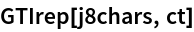Out=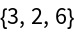Quantitative analysis. To obtain the spectrum of Ho/Pt(111) quantitatively we formulate an effective crystal field Hamiltonian using GTCrystalField. To apply Stevens operators in the second step, we need a real basis, which is obtained by specifying the option GOHarmonics. We furthermore use a cut-off angular momentum of l = 6. Also, for convenience, we apply a slight redefinition of the standard parameters A[l_,m_] -> B[l,m] r^l.
 In:=Out=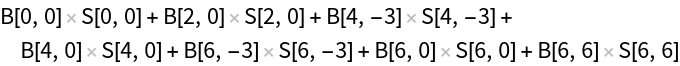The crystal field Hamiltonian is now formulated in terms of crystal field parameters B[l, m] and crystal field operators S[l, m]. We replace the operators S[l, m] by Stevens operators using the command GTStevensOperator. For the B[l, m] we use the respective values from Ref. [Nature 503, 242246 (2013)].
 In:=In:=The Hamiltonian can be diagonalized with the Mathematica command Eigensystem. The eigenvectors are used to calculate the expectation value of the magnetic moment in z - direction <Jz> . The plot reveals a 2-fold degenerate ground state with <Jz>=±8. In agreement with the qualitative analysis, we find 6 two-fold degenerate levels with nonzero <Jz>, as well as five non - degenerate levels with <Jz> = 0.
 In:=In:=In:=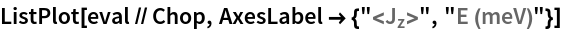Out=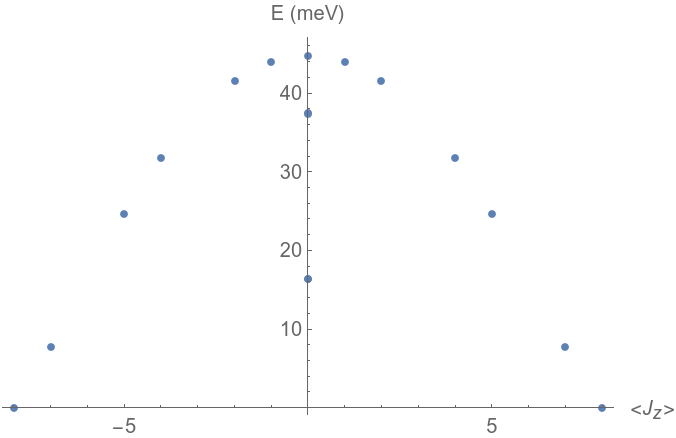In:=Out//TableForm=